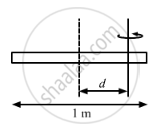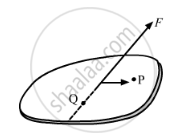Advertisement Remove all ads

# The Moment of Inertia of a Uniform Rod of Mass 0⋅50 Kg and Length 1 M is 0⋅10 Kg-m2 About a Line Perpendicular to the Rod. Find the Distance of this Line from the Middle Point of the Rod. - Physics

Sum

The moment of inertia of a uniform rod of mass 0⋅50 kg and length 1 m is 0⋅10 kg-m2about a line perpendicular to the rod. Find the distance of this line from the middle point of the rod.

Advertisement Remove all ads

#### Solution

Given

Length of the rod, l = 1 m

Mass of the rod, m = 0.5 kg

Let the rod moves at a distance d from the centre.

On applying parallel axis theorem, we get

Moment of inertia about that axis,

$I = \left( \frac{m l^2}{12} \right) + m d^2 = 0 . 10$$I = \frac{0 . 5 \times l^2}{12} + 0 . 5 \times d^2 = 0 . 10$

$\Rightarrow \frac{1}{12} + d^2 = 0 . 2$

$\Rightarrow d^2 = 0 . 118$

$\Rightarrow d = 0 . 342\text{ m from the centre}$Concept: Values of Moments of Inertia for Simple Geometrical Objects (No Derivation)
Is there an error in this question or solution?
Advertisement Remove all ads

#### APPEARS IN

HC Verma Class 11, 12 Concepts of Physics 1
Chapter 10 Rotational Mechanics
Q 12 | Page 196
Advertisement Remove all ads
Advertisement Remove all ads
Share
Notifications

View all notifications

Forgot password?